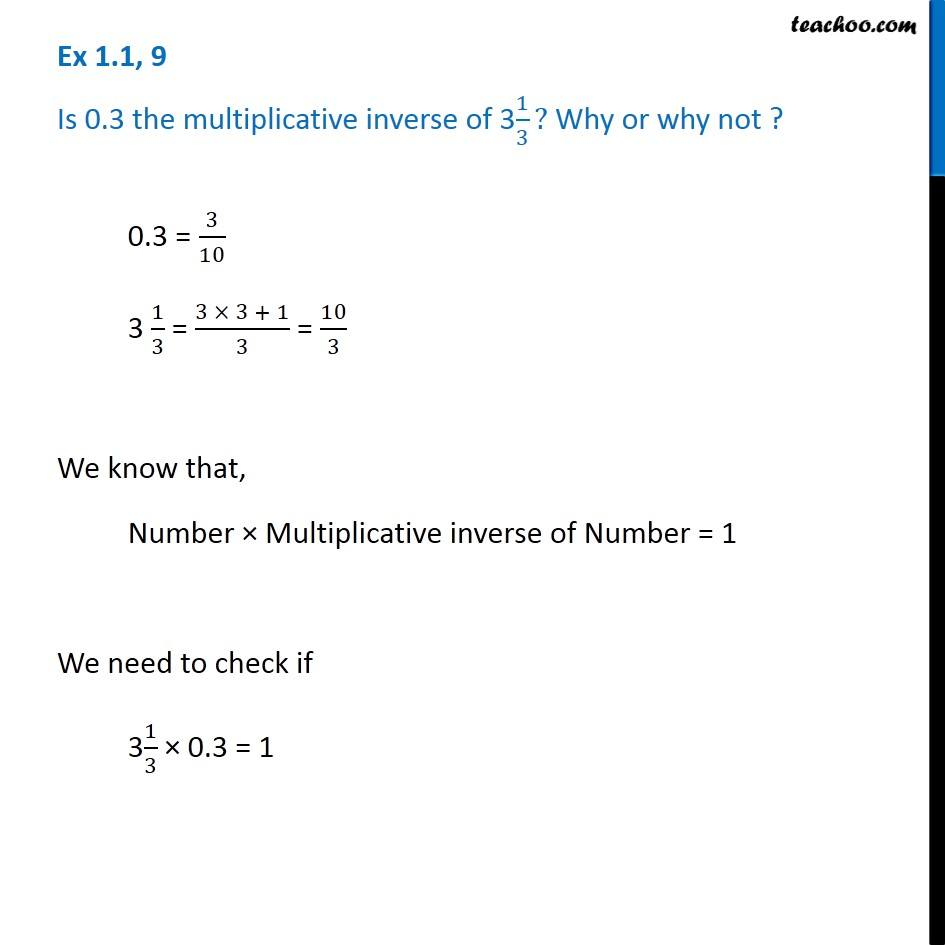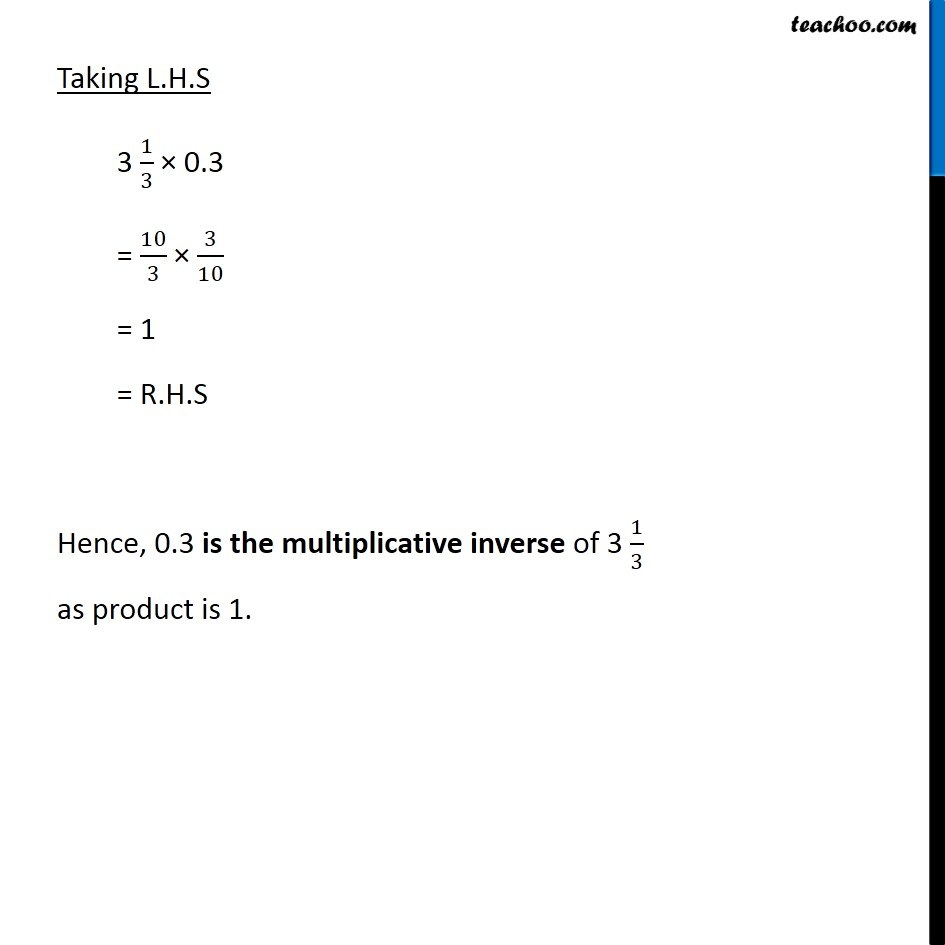Ex 1.1

Chapter 1 Class 8 Rational Numbers
Serial order wiseLearn in your speed, with individual attention - Teachoo Maths 1-on-1 Class

### Transcript

Question 5 Is 0.3 the multiplicative inverse of 31/3? Why or why not ? 0.3 = 3/10 3 1/3 = (3 × 3 + 1)/3 = 10/3 We know that, Number × Multiplicative inverse of Number = 1 We need to check if 31/3 × 0.3 = 1 Taking L.H.S 3 1/3 × 0.3 = 10/3 × 3/10 = 1 = R.H.S Hence, 0.3 is the multiplicative inverse of 3 1/3 as product is 1.